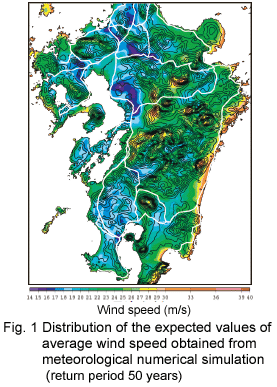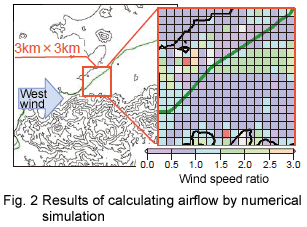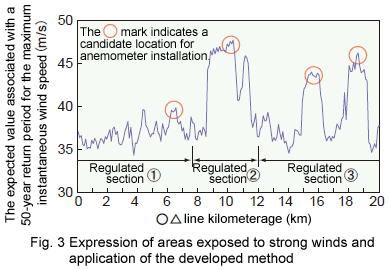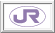1. Development of Method to Pinpoint Areas Exposed to Strong Winds
 Proposal of a method to calculate the expected value associated with the return period of the maximum wind speed along rail wayside. Calculation of the expected value associated with the return period of the maximum instantaneous wind speed in a net of 100-m meshes. Application of a method to determine operation control sections and locations for anemometer installation.For the purpose of discussing sections for operation control in strong winds and locations for anemometer installation, a method of calculating the expected value associated with the return period (referred below simply as the expected value) of the maximum instantaneous wind speed along rail wayside on a net of 100-m meshes was proposed by combining numerical simulation and a statistical technique.

To apply this method, the operation controller first performs meteorological numerical simulation for a net of 3-km meshes, and then calculates the expected value of the average wind speed (equivalent to the average over 10 minutes) in an object area based on the results of the simulation. Fig. 1 shows the results of a calculation for the Kyushu area. After that, the operation controller performs numerical simulation of airflow of rail wayside to examine the effects of the topography on the distribution of wind speeds (Fig. 2). In consideration of the airflow simulation results and the aforementioned expected wind speed value, the distribution of the expected values of the average wind speed is then calculated on a net of 200-m meshes.

The controller also introduces a formula to estimate the ratio of the daily maximum instantaneous wind speed to that of the 10-minute average wind speed (known as the daily gust ratio) with different wind directions on a net of 100-m meshes using a statistical technique. The distribution of the expected values of the average wind speed is then multiplied by the estimated daily gust ratio for different wind directions, and the expected value of the rail wayside maximum wind speed in consideration of the frequencies of the different wind directions that occur is calculated on a net of 100-m meshes.

Using the distribution of the expected values of wayside maximum instantaneous wind speed obtained by this method, it is possible to determine sections for operation control and locations for anemometer installation (Fig. 3).R&D > Major Results of Research and Development in Fiscal 2006 > I Safety/ReliabilityRTRI HOME